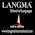# SCC Education

## Trigonometry important questions,multiple angles,conditional identities,relation between sides and interior angles,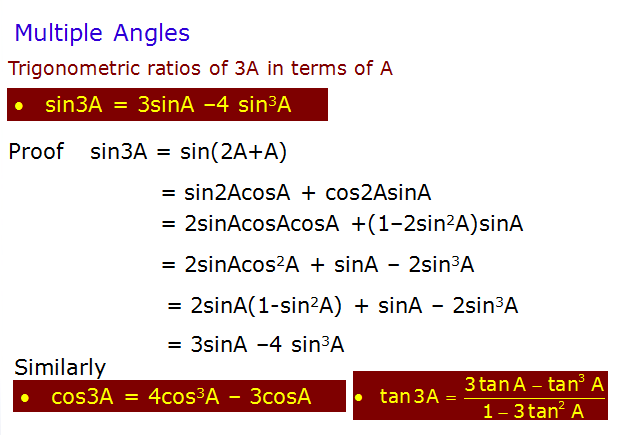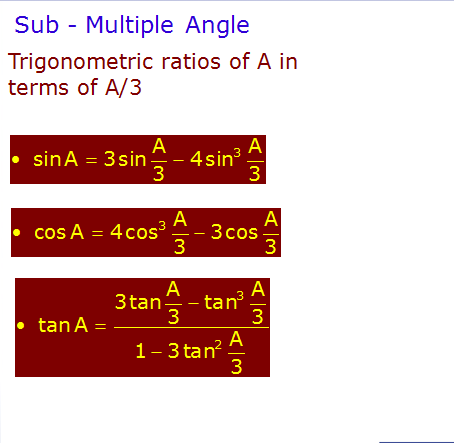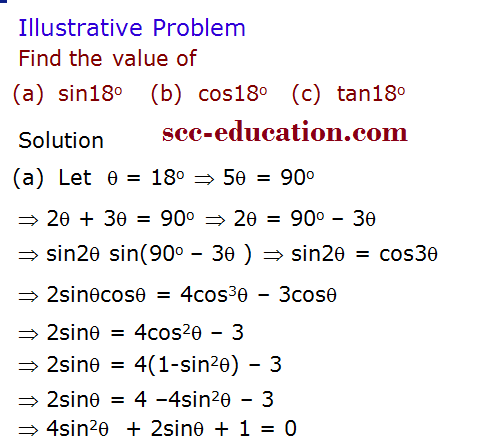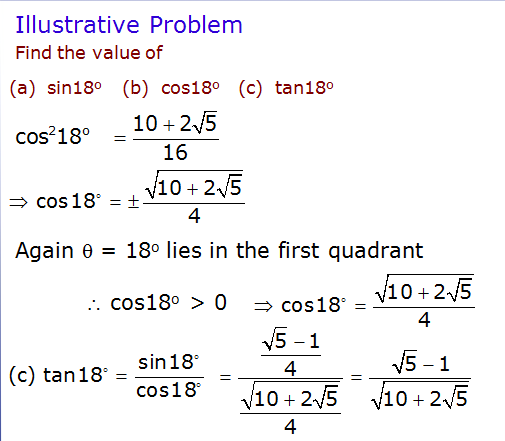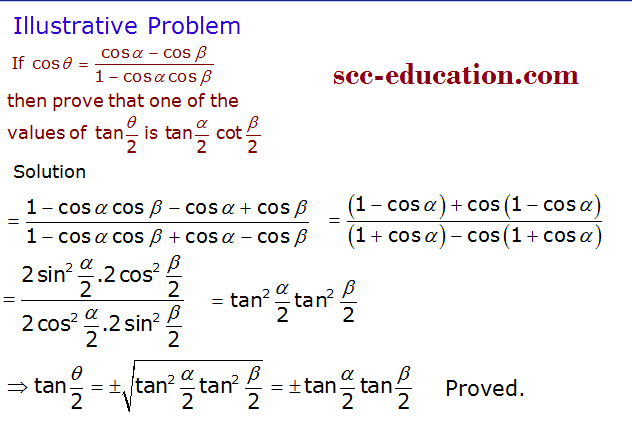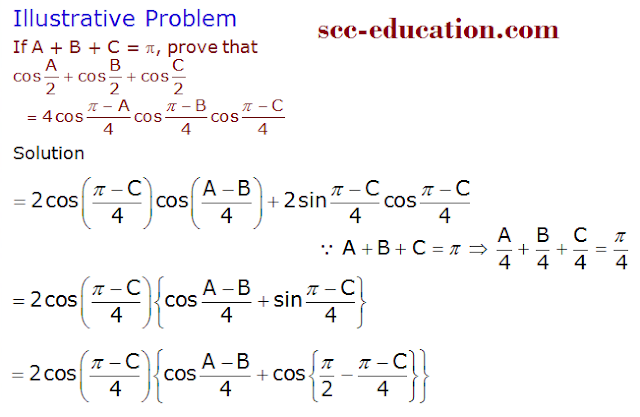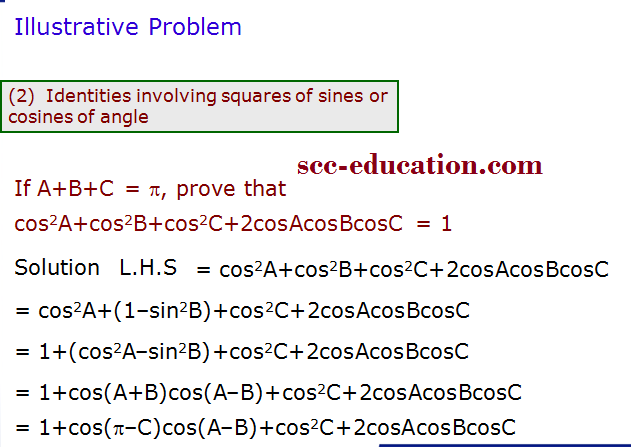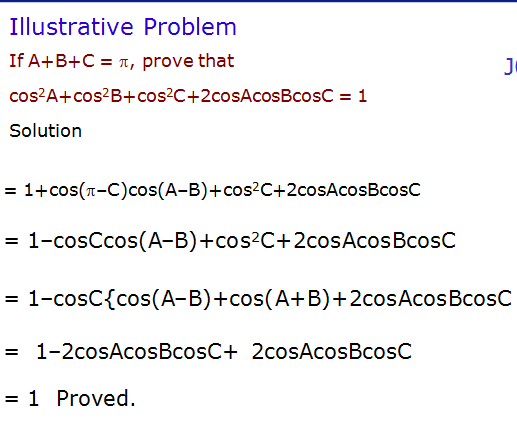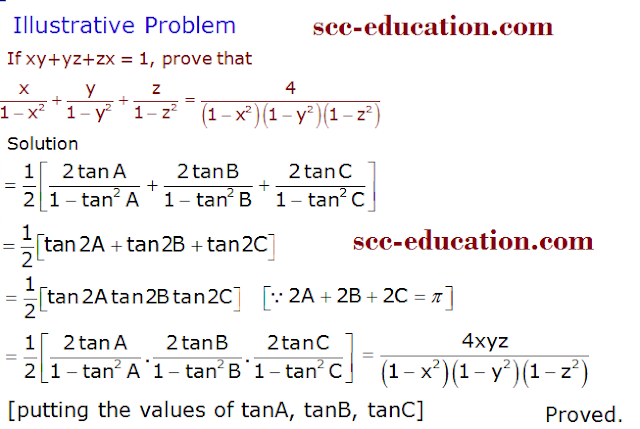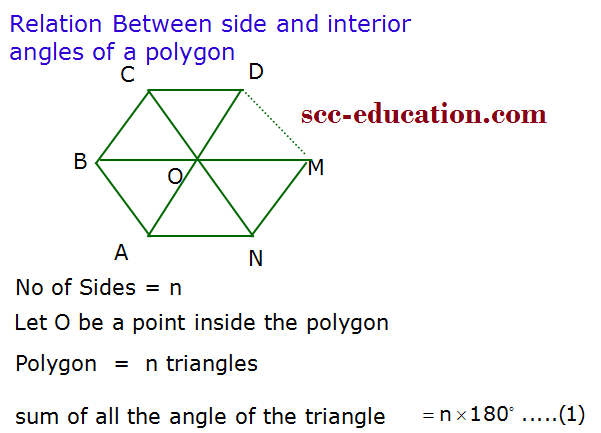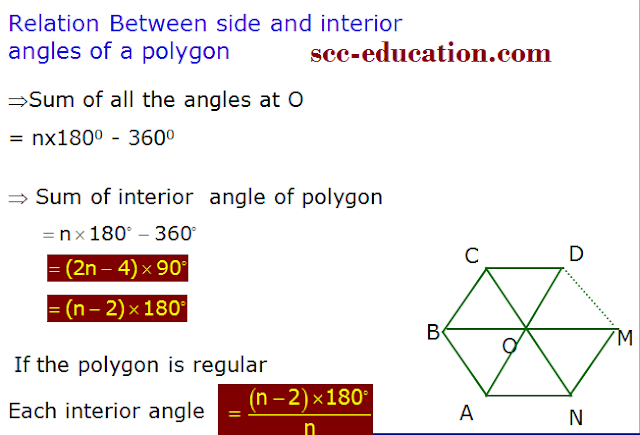Series-formulas

The-binomial-theorem

solved-problems-on-limits-and-continuity

geometric-progressions

Basic-point-formulas-distance-midpoint

Two-point-form-normal-form parametric

limit-questions

set-relation-function-part1

permutation-combination
straight-lines

Three-diamensional-geometry

Trignometry

hyperbola

1.2.3.